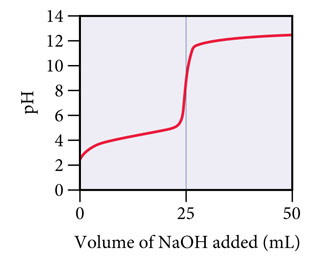# Problem: A weak unknown monoprotic acid is titrated with a strong base. The titration curve is shown below.Find Ka for the unknown acid.

###### FREE Expert Solution

At half-equivalence point:  pH = pK

$\overline{){{\mathbf{pK}}}_{{\mathbf{a}}}{\mathbf{=}}{\mathbf{-}}{{\mathbf{logK}}}_{{\mathbf{a}}}}\phantom{\rule{0ex}{0ex}}\phantom{\rule{0ex}{0ex}}\overline{){{\mathbf{K}}}_{{\mathbf{a}}}{\mathbf{=}}{{\mathbf{10}}}^{\mathbf{-}\mathbf{p}{\mathbf{K}}_{\mathbf{a}}}}$

88% (391 ratings)###### Problem Details

A weak unknown monoprotic acid is titrated with a strong base. The titration curve is shown below.Find Ka for the unknown acid.